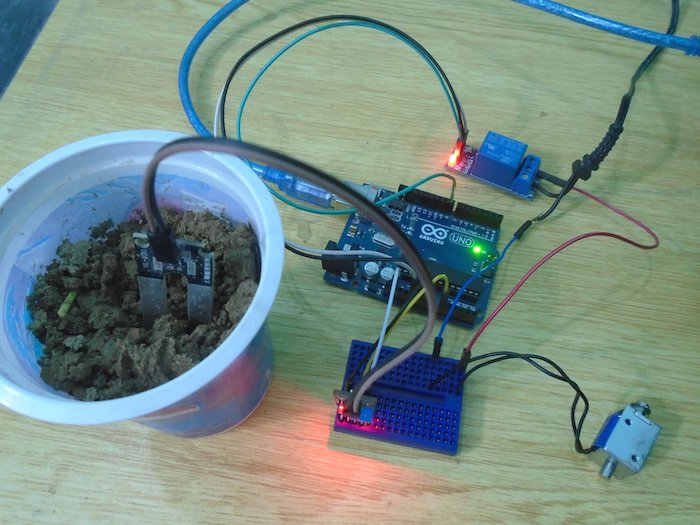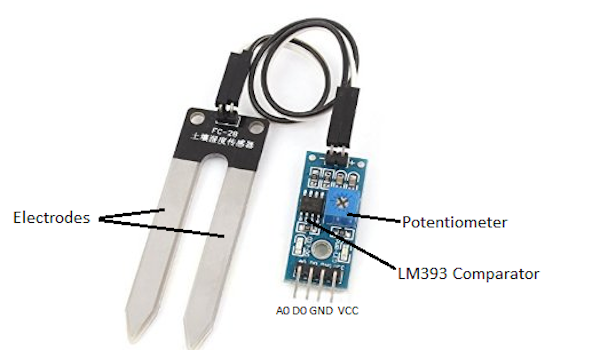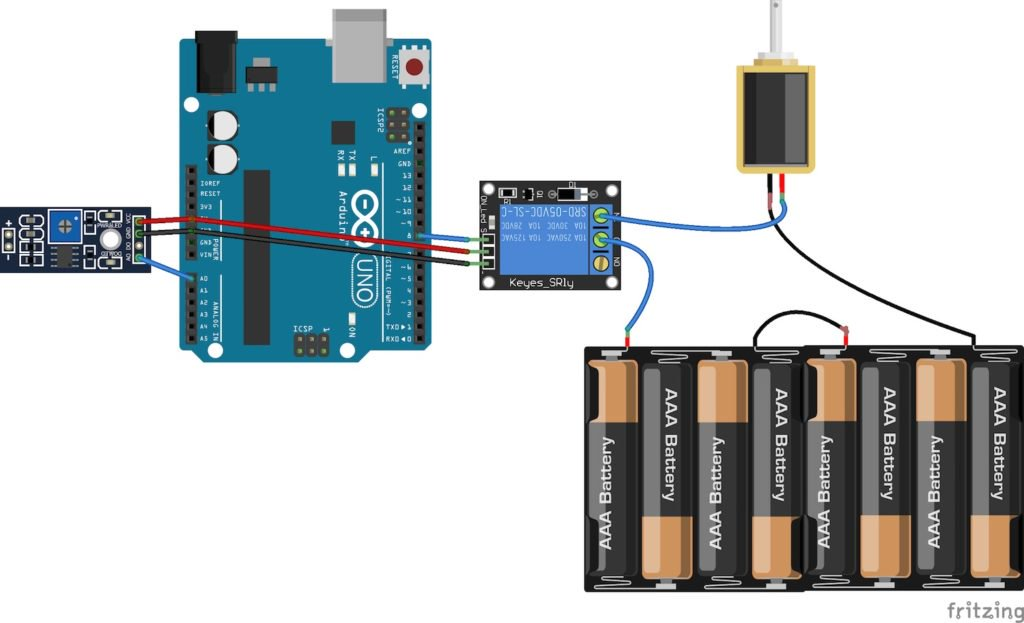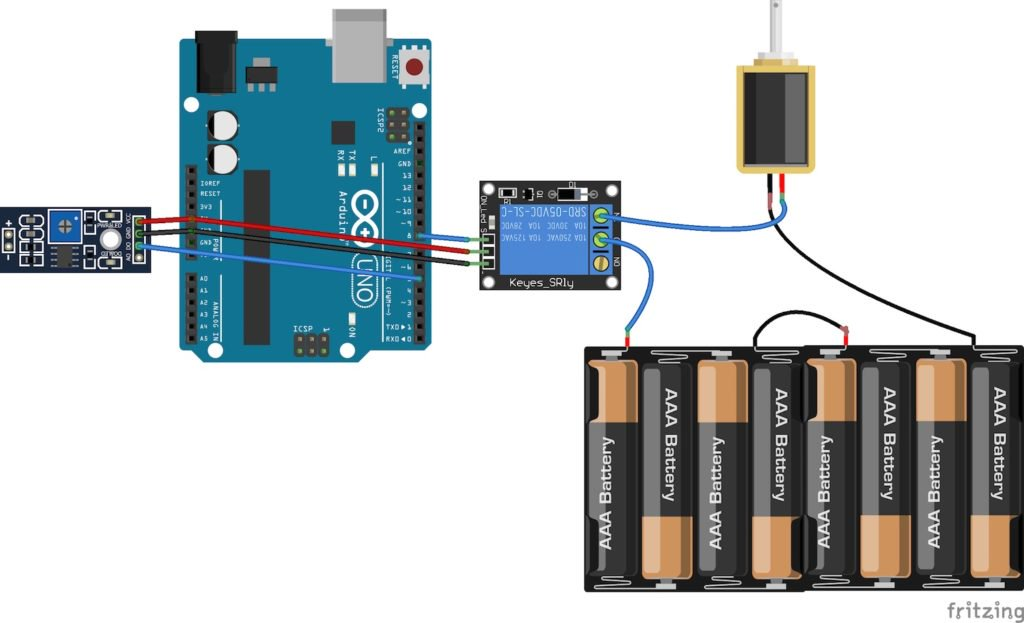Arduino

# How to Test Soil With Arduino and an FC-28 Moisture SensorMarch 23, 2017 by A .
ShareInterface an Arduino with an FC-28 soil moisture sensor to determine if your soil needs water.

## Hardware

 1 ASX00004

In this article, we are going to interface an FC-28 soil moisture sensor with an Arduino. This sensor measures the volumetric content of water in soil and gives us the moisture level. The sensor gives us both analog and digital output. We are going to connect it in both modes.The Arduino soil sensor

### How Does the Arduino Soil Sensor Work?

The soil moisture sensor consists of two probes that are used to measure the volumetric content of water. The two probes allow the current to pass through the soil, which gives the resistance value to measure the moisture value.

When there is water, the soil will conduct more electricity, which means that there will be less resistance. Dry soil conducts electricity poorly, so when there is less water, then the soil will conduct less electricity, which means that there will be more resistance.

This sensor can be connected in analog and digital modes. First, we will connect it in analog mode, and then digital.

### Specifications

The specifications of the FC-28 soil moisture sensor are as follows:
• Input Voltage: 3.3–5V
• Output Voltage: 0–4.2V
• Input Current: 35mA
• Output Signal: both analog and digital

### Pin-out

The FC-28 soil moisture sensor has four pins:
• VCC: Power
• A0: Analog Output
• D0: Digital Output
• GND: Ground
The module also contains a potentiometer, which will set the threshold value. This threshold value will be compared by the LM393 comparator. The output LED will light up and down according to this threshold value.### Analog Mode

To connect the sensor in the analog mode, we will need to use the analog output of the sensor. When taking the analog output from the soil moisture sensor FC-28, the sensor gives us a value from 0 to 1023. The moisture is measured in percentages, so we will map these values from 0 to 100, and then show them on the serial monitor. You can set different ranges of the moisture values and turn the water pump on or off according to it.

### Circuit Diagram

Connect the FC-28 soil moisture sensor to the Arduino as follows:
• VCC of the FC-28 to 5V of the Arduino
• GND of the FC-28 to GND of the Arduino
• A0 of the FC-28 to A0 of the Arduino### Analog Code

```int sensor_pin = A0;
int output_value ;

void setup() {
Serial.begin(9600);
delay(2000);
}

void loop() {

output_value = map(output_value,550,0,0,100);
Serial.print("Mositure : ");
Serial.print(output_value);
Serial.println("%");
delay(1000);
}```

### Code Explanation

First of all, we have defined two variables: one for the soil moisture sensor pin and the other for storing the output of the sensor.
```int sensor_pin = A0; // Soil Sensor input at Analog PIN A0
int output_value ;```

In the setup function, the “Serial.begin(9600)” command will help in communication between the Arduino and serial monitor. Then, we will print “Reading From the Sensor ...” on the serial monitor.

```void setup() {
Serial.begin(9600);
delay(2000);
}```
In the loop function, we will read from the sensor analog pin and will store the values in the “output_ value” variable. Then, we will map the output values to 0–100, because the moisture is measured in percentages. When we took the readings from the dry soil, the sensor value was 550, and in the wet soil, the sensor value was 10. We mapped these values to get the moisture. After that, we printed these values on the serial monitor.
```void loop() {
output_value = map(output_value,550,10,0,100);
Serial.print("Mositure : ");
Serial.print(output_value);
Serial.println("%");
delay(1000);
}```

### Digital Mode

To connect the soil moisture sensor FC-28 in the digital mode, we will connect the digital output of the sensor to the digital pin of the Arduino. The sensor module contains a potentiometer, which is used to set the threshold value. The threshold value is then compared with the sensor output value using the LM393 comparator, which is placed on the sensor module. The LM393 comparator compares the sensor output value and the threshold value, and then gives us the output through the digital pin. When the sensor value is greater than the threshold value, the digital pin will give us 5V, and the LED on the sensor will light up. When the sensor value will be less than this threshold value, the digital pin will give us 0V and the light will go down.

### Circuit Diagram

The connections for the FC-28 soil moisture sensor and the Arduino in digital mode are as follows:
• VCC of FC-28 to 5V of Arduino
• GND of FC-28 to GND of Arduino
• D0 of FC-28 to pin 12 of Arduino
• LED positive to pin 13 of Arduino
• LED negative to GND of Arduino### Digital Code

```int led_pin =13;
int sensor_pin =8;
void setup() {
pinMode(led_pin, OUTPUT);
pinMode(sensor_pin, INPUT);
}
void loop() {
digitalWrite(led_pin, HIGH);
} else {
digitalWrite(led_pin, LOW);
delay(1000);
}
}

```

### Digital Code Explanation

First of all, we have initialized two variables for connecting the LED pin and the sensor's digital pin.
```int led_pin =13;
int sensor_pin =8;

```
In the setup function, we have declared the LED pin as the output pin, because we will power the LED through that pin. We declared the sensor pin as the input pin, because the Arduino will take the values from the sensor through that pin.

```void setup() {
pinMode(led_pin, OUTPUT);
pinMode(sensor_pin, INPUT);
}```

In the loop function, we have read from the sensor pin. If the output value of the sensor is higher than the threshold value, then the digital pin will be high and the LED will light up. If the sensor value will be lower than the threshold value, then the LED will go down.

```void loop() {
digitalWrite(led_pin, HIGH);
} else {
digitalWrite(led_pin, LOW);
delay(1000);
}
}```

### AuthorA .

For custom projects, hire me at https://www.freelancer.pk/u/Muhammadaqibdutt